Next: Selecting the Optimal P3P Up: Inverse 3D Projection Previous: Inverse 3D Projection

## P3P - Fitting the 3D Model to a 2D Image

If the deformation variable, sy is held constant, only 6 degrees of freedom remain in Equationand an exact solution which involves 6 distances can be found to the problem of fitting to three 2D points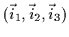. At this stage, we choose to usewhich correspond to the eyes and the nose to perform the fitting since we consider their loci to be more accurate and more stable than the mouth's locus. The fitting then reduces to the well-known Perspective-3-Points (P3P) problem . A direct solution is possible due to the 1-to-1 nature of the problem. There exist more complex solutions capable of finding optimal fits for over-constrained multi-point problems ,  and ; however, we shall defer the fitting to the 4th point (the mouth) for now.

It is important at this stage to consider perspective. In 3D rendering, perspective generally enhances the realism of a scene. Buildings and geometric objects appear to taper off or shrink as they move away from the user in distance. If, however, the size of the object in depth is relatively small, the perspective effect is negligible. The effect of perspective on face images is subtle to the human eye and it is thus possible to render 3D face data without such perspective computations. We shall utilize this simplification and approach the solution of fitting to three points using the Weak-Perspective-3-Points (WP3P) technique . A brief summary of the computation of the solution to WP3P is presented here, but for an in-depth analysis of the derivation the reader should consult .

Observe Figurewhich depicts the desired, scaled orthographic projection of the model upon the image plane. The intra-point distances in the figure are (R01, R02, R12) for the model and (d01,d02,d12) for the image object. The overall scaling the model needs to undergo to fit the image is defined as s. The vertical heights from the image plane of the aligned model's two vertices are (H1,H2) before scaling or (h1,h2) after scaling. The parameters in Figureare computed using Equations,and: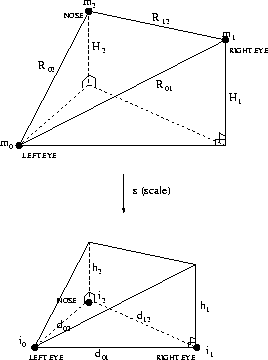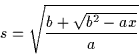(4.3)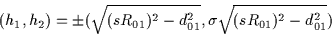(4.4)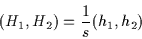(4.5)

 a = (R01+R02+R12) (-R01+R02+R12) (R01-R02+R12) (R01+R02-R12) (4.6)

 b = d012(-R012+R022+R122) + d022(R012-R022+R122) + d122(R012+R022-R122) (4.7)

 c = (d01+d02+d12) (-d01+d02+d12) (d01-d02+d12) (d01+d02-d12) (4.8)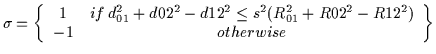(4.9)

We then solve for the rotation matrix using the intermediate matrices A and B using Equationand Equation: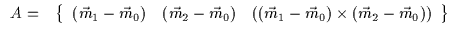(4.10)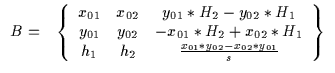(4.11)

where x01, x02, y01, y02 are 2D coordinates relative to a coordinate system centered at the position of the left eye,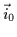:

 x01=i1x - i0x (4.12) x02=i2x - i0x (4.13) y01=i1y - i0y (4.14) y02=i2y - i0y (4.15)

The rotation matrix, R, can then be computed using Equation:

 R= BA-1 (4.16)

The translation vector, t, is computed simply by translating the centered coordinate system to the position of. The translation in the depth dimension is irrelevant and can be omitted since scaling is directly controlled by s (orthographic projection is not scaled by depth):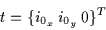(4.17)

Once the values of R and t have been determined, any 3D model point can be transformed and the points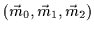will align with the image points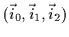. The transformation from model point to image point is thus: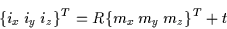(4.18)

Of course, the iz value is only a relative measurement of the depth of the model point. It is useful, however, for keeping track of the relative depth of the model point with respect to other model points.

Note that this is a direct solution of the WP3P problem save for one ambiguity: the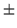value in the computation of (h1,h2) in Equation. This ambiguity allows two possible alignments of the model to the image points. The 3D face can either line up by facing towards or away from the viewer. Of course, we know that the face is projecting onto the image from behind the image plane and is facing the viewer (or camera-man''). Thus we select either + or - in Equationto assure that the 3D model is actually behind the image plane and is facing towards the camera. This ambiguity is resolved by computing the normal of the nose. In other words, a vector protruding from the nose on the model is introduced and undergoes the transformation in Equation. We begin by calculating Equationwith a '+'. We note the relative depth value of the vector iz. If the vector is pointing away from the viewer (its tip is farther from the image plane than its base or, equivalently, has a larger iz value) then the model is pointing away from the camera and we repeat the computation with a '-' in Equation.

The end result is a mapping from the 3D model to the image which lines up the eyes and the nose optimally.Next: Selecting the Optimal P3P Up: Inverse 3D Projection Previous: Inverse 3D Projection
Tony Jebara
2000-06-23